#Function Repository Resource:

# SimplifyRepeatedSubexpressions

Replace repeated subexpressions in an expression with new symbols

Contributed by: Jon McLoone
 ResourceFunction["SimplifyRepeatedSubexpressions"][expr] returns a list of the expr with repeated subexpressions removed, along with the replacement rules used to rewrite them in simpler form.

## Examples

### Basic Examples

Find the repeated term in the expression (a+b+c)3+(a+b+c)2:

 In:=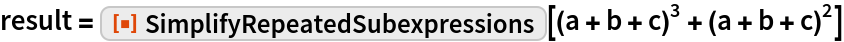Out=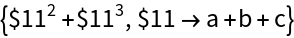Reconstruct the original expression from the decomposed form:

 In:=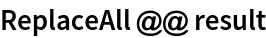Out=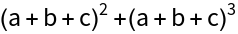### Options

Finding many small common subexpressions may not be helpful:

 In:=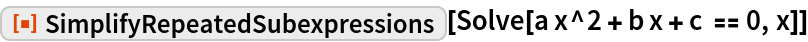Out=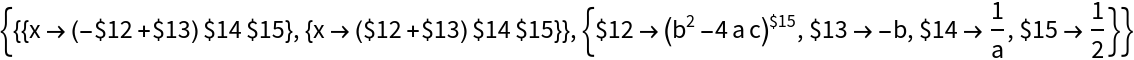Find only the largest with "MinLeafCount":

 In:=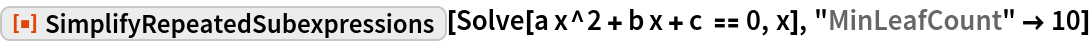Out=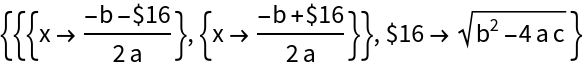### Applications

Remove the discriminant from the quadratic solution equations:

 In:=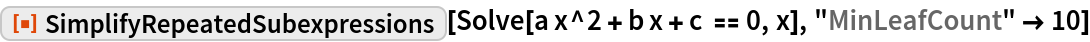Out=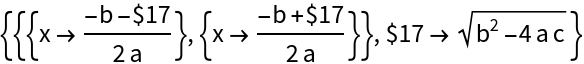### Properties and Relations

Reconstruct the original expression from the decomposed form by applying ReplaceAll:

 In:=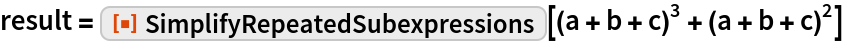Out=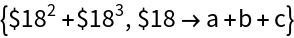In:=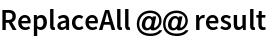Out=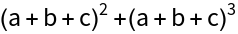### Possible Issues

The FullForm of subexpressions must match; in this case no match is found:

 In:=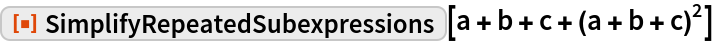Out=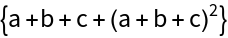This is because the outermost Plus has four arguments and not just the a, b and c:

 In:=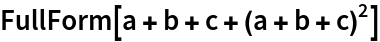Out=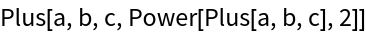If the input expression contains elements with HoldAll, HoldRest or HoldFirst, the contents will evaluate during the search:

 In:=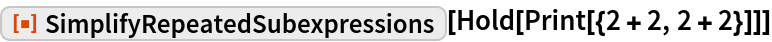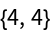Out=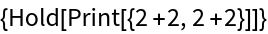### Neat Examples

Simplify the order three polynomial solution:

 In:=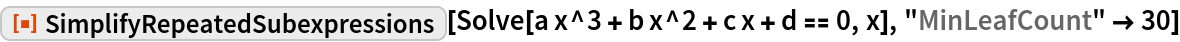Out=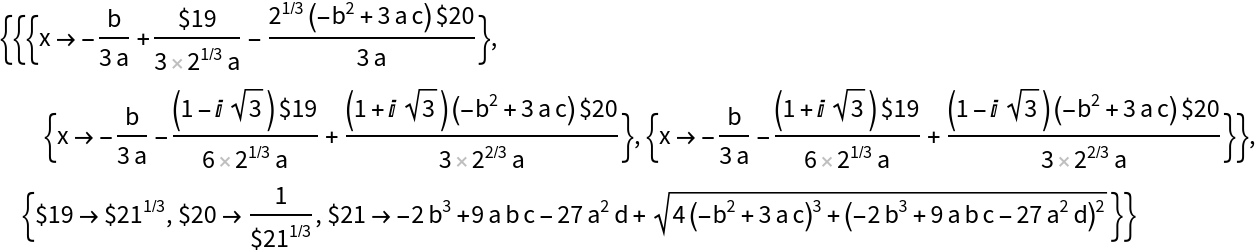And the order four polynomial solution:

 In:=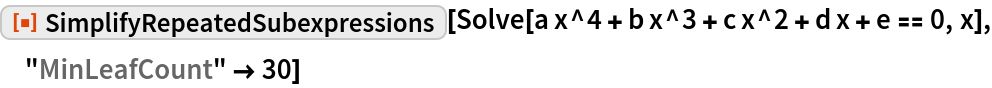Out=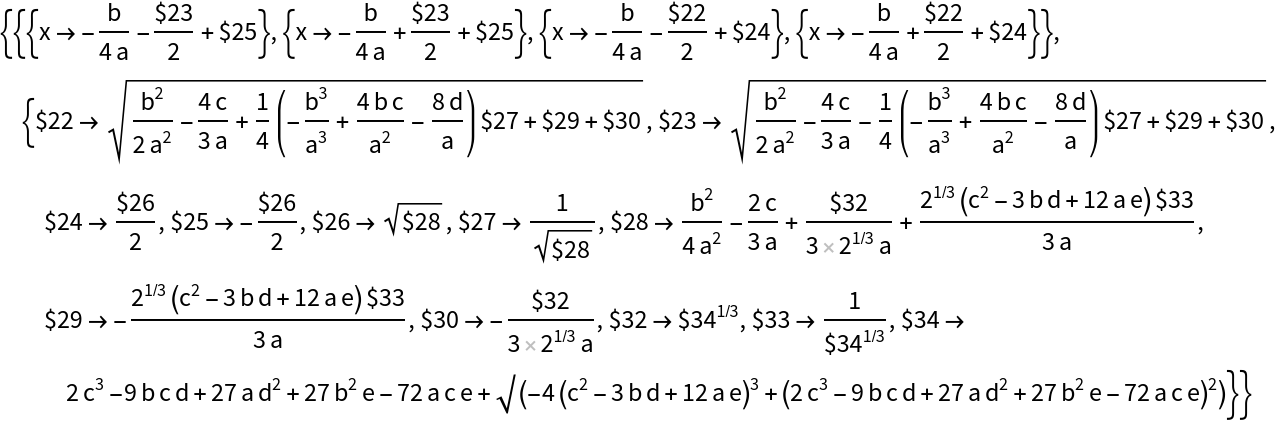## Requirements

Wolfram Language 11.3 (March 2018) or above